NEET  >  Test: Semiconductor Electronics

# Test: Semiconductor Electronics

Test Description

## 30 Questions MCQ Test Physics Class 12 | Test: Semiconductor Electronics

Test: Semiconductor Electronics for NEET 2023 is part of Physics Class 12 preparation. The Test: Semiconductor Electronics questions and answers have been prepared according to the NEET exam syllabus.The Test: Semiconductor Electronics MCQs are made for NEET 2023 Exam. Find important definitions, questions, notes, meanings, examples, exercises, MCQs and online tests for Test: Semiconductor Electronics below.
Solutions of Test: Semiconductor Electronics questions in English are available as part of our Physics Class 12 for NEET & Test: Semiconductor Electronics solutions in Hindi for Physics Class 12 course. Download more important topics, notes, lectures and mock test series for NEET Exam by signing up for free. Attempt Test: Semiconductor Electronics | 30 questions in 30 minutes | Mock test for NEET preparation | Free important questions MCQ to study Physics Class 12 for NEET Exam | Download free PDF with solutions
 1 Crore+ students have signed up on EduRev. Have you?
Test: Semiconductor Electronics - Question 1

### Basic materials used in the present solid state electronic devices like diode, transistor, ICs, etc are

Detailed Solution for Test: Semiconductor Electronics - Question 1

Explanation:Semiconductors are materials that have electrical conductivity between conductors such as most metals and nonconductors or insulators like ceramics. Semiconductor material is used in the manufacturing of electrical components and used in electronic devices such as transistors and diodes.Semiconductors are the basic materials used in the present solid state electronic devices like diode, transistor, ICs etc

Test: Semiconductor Electronics - Question 2

### Depletion region (space charge) is formed because

Detailed Solution for Test: Semiconductor Electronics - Question 2

Explanation:The depletion region, also called depletion layer, depletion zone, junction region, space charge region or space charge layer, is an insulating region within a conductive, doped semiconductor material where the mobile charge carriers have been diffused away, or have been forced away by an electric field. The only elements left in the depletion region are ionized donor or acceptor impurities.The depletion region is so named because it is formed from a conducting region by removal of all free charge carriers, leaving none to carry a current.

Test: Semiconductor Electronics - Question 3

### The conductivity of P – type semiconductor is due to

Detailed Solution for Test: Semiconductor Electronics - Question 3

A P-type semiconductor is formed when a trivalent electron deficient impurities such as boron group elements are doped with intrinsic semiconductor. As the impurities are electron deficient, they take electrons from the valence band creating a number of holes. Due this reason conductivity in P-type semiconductor is mainly due to holes rather than electrons.

Test: Semiconductor Electronics - Question 4

In an N-P-N transistor, P-type crystal is

Detailed Solution for Test: Semiconductor Electronics - Question 4

Explanation:When the p-type crystal is grown between relatively wide sections of n-type crystals then the transistor is called NPN transistor.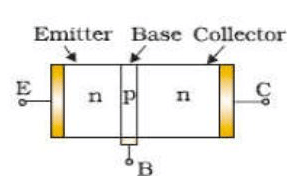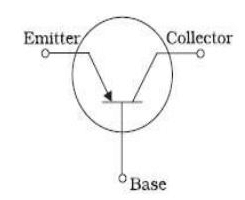Test: Semiconductor Electronics - Question 5

Domestic power supply in India is

Detailed Solution for Test: Semiconductor Electronics - Question 5

Concept:

• As per Indian standard for domestic power supply we use A.C current, which is also known as alternating current since they change its polarity at a certain interval of time.
• Now the two principal properties of the A.C electric power supply are Voltage and frequency.
• And we use 220 V and 50 Hz power supply for our common domestic use
• Where 220 V is the potential difference and 50 Hz is its frequency.

Explanation:

The voltage and frequency of domestic power supply used in India are 220 volt and 50 Hz respectively

Test: Semiconductor Electronics - Question 6

In the case of metals the valence and conduction bands have

Detailed Solution for Test: Semiconductor Electronics - Question 6

Explanation:The materials can be classified by the energy gap between their valence band and the conduction band. The valence band is the band consisting of the valence electron, and the conduction band remains empty. Conduction takes place when an electron jumps from valence band to conduction band and the gap between these two bands is forbidden energy gap. Wider the gap between the valence and conduction bands, higher the energy it requires for shifting an electron from valence band to the conduction band.In the case of conductors, this energy gap is absent or in other words conduction band, and valence band overlaps each other. Thus, electron requires minimum energy to jump from valence band. The typical examples of conductors are Silver, Copper, and Aluminium.In insulators, this gap is vast. Therefore, it requires a significant amount of energy to shift an electron from valence to conduction band. Thus, insulators are poor conductors of electricity. Mica and Ceramic are the well-known examples of insulation material.Semiconductors, on the other hand, have an energy gap which is in between that of conductors and insulators. This gap is typically more or less 1 eV, and thus, one electron requires energy more than conductors but less than insulators for shifting valence band to conduction band.

Test: Semiconductor Electronics - Question 7

In a p-n junction, as the diffusion process continues the width of the depletion zone

Detailed Solution for Test: Semiconductor Electronics - Question 7

In reverse biasing, the positive terminal of the battery is connected to the n-type whereas the negative terminal is connected to the p-type junction. So the positive terminal tends to pull the electrons (near to the depletion layer) in n-type towards itself whereas the negative terminal pulls the holes towards itself which results in an increase in the width of the depletion layer.

Test: Semiconductor Electronics - Question 8

An N-type Ge is obtained on doping the Ge- crystal with

Detailed Solution for Test: Semiconductor Electronics - Question 8

Explanation:The addition of pentavalent impurities such as antimony, arsenic or phosphorous contributes free electrons, greatly increasing the conductivity of the intrinsic semiconductor.

Test: Semiconductor Electronics - Question 9

A p- type semiconductor can be obtained by adding

Detailed Solution for Test: Semiconductor Electronics - Question 9

Explanation:In P-type doping, boron or gallium is the dopant. Boron and gallium each have only three outer electrons. When mixed into the silicon lattice, they form "holes" in the lattice where a silicon electron has nothing to bond to. The absence of an electron creates the effect of a positive charge, hence the name P-type.Holes can conduct current. A hole happily accepts an electron from a neighbor, moving the hole over a space. P-type silicon is a good conductor.

Test: Semiconductor Electronics - Question 10

In a semiconductor crystal, if current flows due to breakage of crystal bonds, then the semiconductor is called

Detailed Solution for Test: Semiconductor Electronics - Question 10

In the case of an intrinsic semiconductor (say Si) where each Si is having 4 outermost electrons, its crystal structure consists of making 4 covalent bonds with 4 neighbouring Si atoms. Each bond consists of two electrons.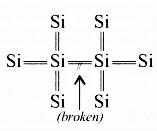Now if one of the bonds gets broken due to some reason (collisions or high temperature) then one electron gets free and it will be having sufficient energy to cross the band gap and be ready for conduction- So in intrinsic semiconductors, current flows due to breakage of crystal bonds.

Test: Semiconductor Electronics - Question 11

Hole is a vacancy or lack of an electron and can travel through the semiconductor material. It can

Detailed Solution for Test: Semiconductor Electronics - Question 11

Explanation:Holes and electrons are the two types of charge carriers responsible for current in semiconductor materials.Holes in a metal or semiconductor crystal lattice can move through the lattice as electrons can, and act similarly to positively-charged particles. They play an important role in the operation of semiconductor devices such as transistors, diodes and integrated circuits. However they are not actually particles, but rather quasiparticles; they are different from the positron, which is the antiparticle of the electron.

Test: Semiconductor Electronics - Question 12

Which of the following is not a semiconductor?

Detailed Solution for Test: Semiconductor Electronics - Question 12

Explanation:Materials that have the resistance levels between those of a conductor and an insulator are referred to as semiconductors.They are quite common, found in almost all electronic devices. Good examples of semiconductor materials are germanium, selenium, and silicon.Radium is a chemical element with symbol Ra and atomic number 88. It is the sixth element in group 2 of the periodic table, also known as the alkaline earth metals.

Test: Semiconductor Electronics - Question 13

Majority current carriers in N – types are

Detailed Solution for Test: Semiconductor Electronics - Question 13

Explanation:The more abundant charge carriers are called majority carriers, which are primarily responsible for current transport in a piece of semiconductor. In n-type semiconductors they are electrons, while in p-type semiconductors they are holes.

Test: Semiconductor Electronics - Question 14

The depletion layer in the p-n junction is caused

Detailed Solution for Test: Semiconductor Electronics - Question 14

When a p−n junction is formed, some of the free electrons in the n region diffuse across the junction and combine with holes to form negative ions. In doing so they leave behind positive ions at the donor impurity sites. Similarly, holes from p side diffuse to the n side and thus form a layer called diffusion layer at the junction.
So the depletion region is caused by the diffusion of charge carriers.

Test: Semiconductor Electronics - Question 15

When n –type of semiconductor is heated

Detailed Solution for Test: Semiconductor Electronics - Question 15

Explanation:Due to heating, when a free electron is produced then simultaneously a hole is also produced.

Test: Semiconductor Electronics - Question 16

In a pure, or intrinsic, semiconductor, valence band holes and conduction-band electrons are always present

Detailed Solution for Test: Semiconductor Electronics - Question 16

Explanation:An intrinsic semiconductor, also called an undoped semiconductor or i-type semiconductor, is a pure semiconductor without any significant dopant species present. The number of charge carriers is therefore determined by the properties of the material itself instead of the amount of impurities. In intrinsic semiconductors the number of excited electrons and the number of holes are equal: n = p.

Test: Semiconductor Electronics - Question 17

In a semiconductor, the forbidden energy gap between the valance band and the conduction band is of the order of

Detailed Solution for Test: Semiconductor Electronics - Question 17

Explanation:The material which has electrical conductivity between that of a conductor and an insulator is called as semiconductor. Silicon, germanium and graphite are some examples of semiconductors.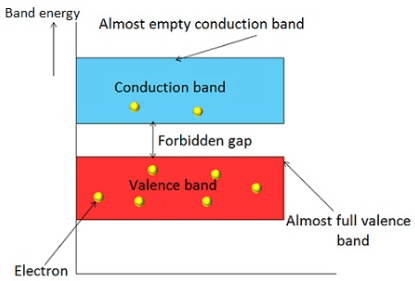In semiconductors, the forbidden gap between valence band and conduction band is very small. It has a forbidden gap of about 1 electron volt (eV).

Test: Semiconductor Electronics - Question 18

A solid having uppermost energy – band partially filled with electrons is called

Detailed Solution for Test: Semiconductor Electronics - Question 18

Conductor is an object or type of material that allows the flow of an electrical current in one more directions. A metal wire is a common electrical conductor. In metals such as copper or aluminium, the mobile charged particles are welcome.

Test: Semiconductor Electronics - Question 19

Doping of semiconductor is the process of

Detailed Solution for Test: Semiconductor Electronics - Question 19

Explanation:Doping is the process of adding impurities to intrinsic semiconductors to alter their properties. Normally Trivalent and Pentavalent elements are used to dope Silicon and Germanium. When an intrinsic semiconductor is doped with Trivalent impurity it becomes a P-Type semiconductor.

Test: Semiconductor Electronics - Question 20

Hole is

Detailed Solution for Test: Semiconductor Electronics - Question 20

Concept of holes in the semiconductor:

1. When an electron is removed from a covalent bond, it leaves a vacancy behind. An electron from a neighbouring atom can move into this vacancy, leaving the neighbour with a vacancy. In this way the vacancy formed is called a hole (or cotter), and can travel through the material and serve as an additional current carriers.
2. A hole is considered as a seat of positive charge, having magnitude of charge equal to that of an electron.
3. Holes acts as a virtual charge, although there is no physical charge on it.
4. Effective mass of hole is more than an electron.
5. Mobility of hole is less than an electron.
Test: Semiconductor Electronics - Question 21

When a P- N junction is reversed biased, the flow of current across the junction is mainly due to

Detailed Solution for Test: Semiconductor Electronics - Question 21

When p-n junction is reverse biased, the flow of current is due to drifting of minority charge carriers across the junction.

Test: Semiconductor Electronics - Question 22

The main difference between conductors, semiconductors and insulators is because of

Detailed Solution for Test: Semiconductor Electronics - Question 22

Explanation:Forbidden gap plays a major role for determining the electrical conductivity of material. Based on the forbidden gap materials are classified in to three types, they are : Insulators : The forbidden gap between the valence band and conduction band is very large in insulators. The energy gap of insulator is approximately equal to 15 electron volts (eV).Conductors: In a conductor, valence band and conduction band overlap each other. Therefore, there is no forbidden gap in a conductor.Semiconductors: In semiconductors, the forbidden gap between valence band and conduction band is very small. It has a forbidden gap of about 1 electron volt (eV).

Test: Semiconductor Electronics - Question 23

The number of valence electrons in a good conductor is generally

Detailed Solution for Test: Semiconductor Electronics - Question 23

Explanation:The electron theory states that all matter is composed of atoms and the atoms are composed of smaller particles called protons, electrons, and neutrons. The electrons orbit the nucleus which contains the protons and neutrons. It is the valence electrons that we are most concerned with in electricity. These are the electrons which are easiest to break loose from their parent atom. Normally, conductors have three or less valence electrons; insulators have five or more valence electrons; and semiconductors usually have four valence electrons.

Test: Semiconductor Electronics - Question 24

In the middle of the depletion layer of a reverse biased p-n-junction, the

Detailed Solution for Test: Semiconductor Electronics - Question 24

Due to the reverse biasing, the width of the depletion region increases and current flowing through the diode is almost zero. In this case, electric field is almost zero at the middle of the depletion region.

Test: Semiconductor Electronics - Question 25

The output from a full wave rectifier is

Detailed Solution for Test: Semiconductor Electronics - Question 25

A Full Wave Rectifier is a circuit, which converts an ac voltage into a pulsating dc voltage using both half cycles of the applied ac voltage. It uses two diodes of which one conducts during one half cycle while the other conducts during the other half cycle of the applied ac voltage.
During the positive half cycle of the input voltage, diode D1 becomes forward biased and D2 becomes reverse biased.Hence D1 conducts and D2 remains OFF. The load current flows through D1 and the voltage drop across RL will be equal to the input voltage.During the negative half cycle of the input voltage, diode D1 becomes reverse biased and D2 becomes forward biased.Hence D1 remains OFF and D2 conducts. The load current flows through D2 and the voltage drop across RL will be equal to the input voltage.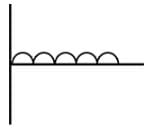Test: Semiconductor Electronics - Question 26

Motion of hole is a convenient way of describing

Detailed Solution for Test: Semiconductor Electronics - Question 26

Explanation:The holes are just the abscence of a electron in a energy band. But its easier to describe the abscence of a electron as a single moving positive charge than it is to describe the motion of all the other electrons in the band.In a band you have N electrons. Remove one of those electrons(by for instance p-doping) and you have N-1 electrons left. Now you can either choose to describe this with the behavior of those N-1 electrons. Or you can choose to describe it as if there is one single hole moving around in the band.So mathematicly the holes behave just like a positivly charged electron.

Test: Semiconductor Electronics - Question 27

When the conductivity of a semiconductor is only due to breaking of the covalent bonds, the semiconductor is called

Detailed Solution for Test: Semiconductor Electronics - Question 27

Explanation:A pure semiconductor is called intrinsic semiconductor, e.g., silicon, germanium. The presence of the mobile charge carriers is the intrinsic property of the material. At room temperature, some covalent bonds are broken and electrons are made free. The absence of electron in the covalent bond form hole.The electrical conduction is by means of mobile electrons and holes. Hole act as positive charge, because it can attract an electron. If some other bond is broken and the electron thus freed fills this hole(vacancy), it seems as though the hole is moving.Actually an electron is travelling in opposite direction. In a pure(intrinsic) semiconductor, the number of holes is equal to the number of free electrons.

Test: Semiconductor Electronics - Question 28

Electrons are forbidden in a band (in a crystal) called the

Detailed Solution for Test: Semiconductor Electronics - Question 28

Explanation:A region of values of energy that electrons in an ideal crystal (without defects) cannot have. In semiconductors the forbidden band separating the valence band and the conduction band is usually considered. In this case the energy difference between the lower level (bottom) of the conduction band and the upper level (ceiling) of the valence band is called the width of the forbidden band.

Test: Semiconductor Electronics - Question 29

The difference in variation of resistance with temperature in a metal and semiconductor is due to

Detailed Solution for Test: Semiconductor Electronics - Question 29

In metals, scattering increases with temperature and hence the flow of electrons is restricted. In semiconductors, the number of charge carriers increases with temperature which overcomes the effect of increasing scattering and hence the resistivity of semiconductors decreases with an increase in temperature but that of metals increases.

Test: Semiconductor Electronics - Question 30

A piece of copper and another of germanium are cooled from room temperature to 80 K. The resistance of

Detailed Solution for Test: Semiconductor Electronics - Question 30

We know, a piece of copper is a metal while that of germanium is a semiconducting material. For metals resistance increases with increase in temperature. The semiconductor has a negative temperature coefficient of resistance. Hence, when it is cooled its resistance increases.

## Physics Class 12

157 videos|452 docs|213 tests
 Use Code STAYHOME200 and get INR 200 additional OFF Use Coupon Code
Information about Test: Semiconductor Electronics Page
In this test you can find the Exam questions for Test: Semiconductor Electronics solved & explained in the simplest way possible. Besides giving Questions and answers for Test: Semiconductor Electronics , EduRev gives you an ample number of Online tests for practice

## Physics Class 12

157 videos|452 docs|213 tests

### How to Prepare for NEET

Read our guide to prepare for NEET which is created by Toppers & the best Teachers